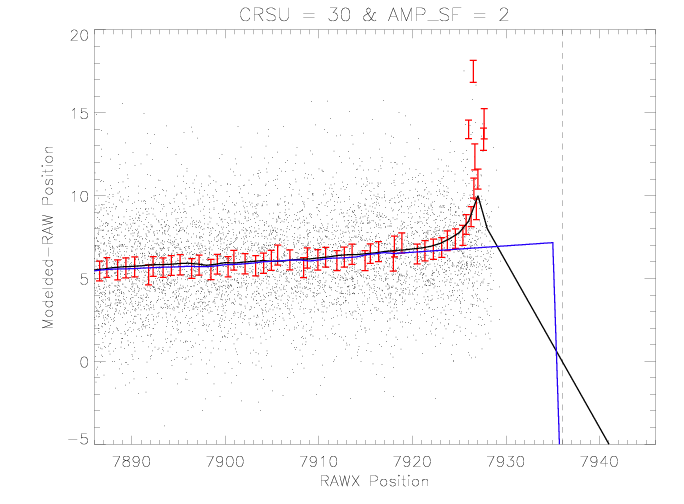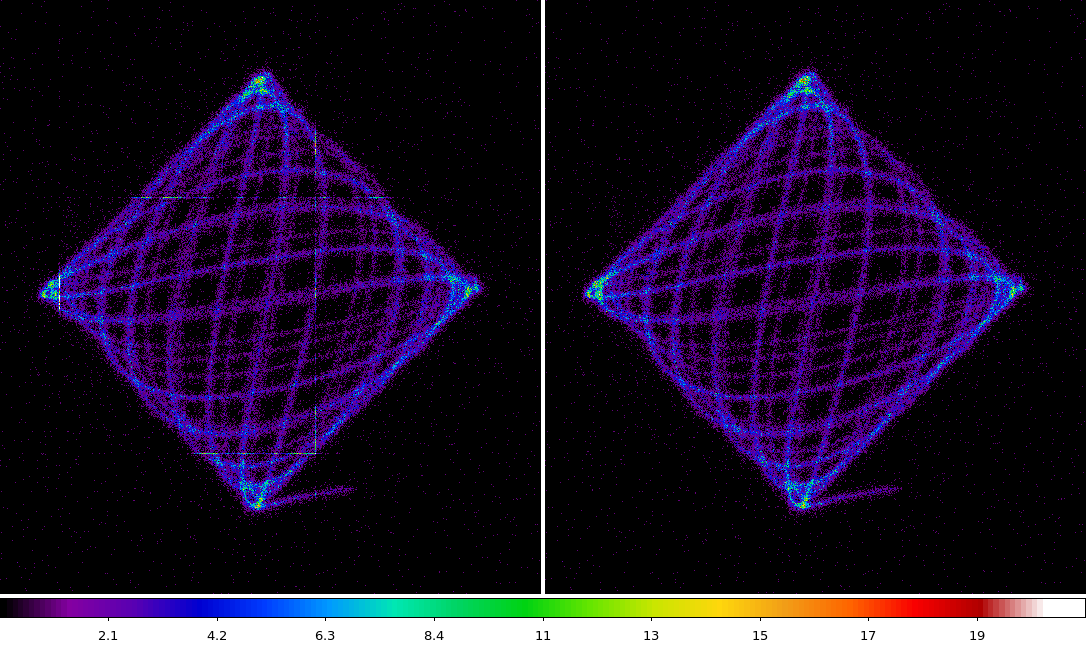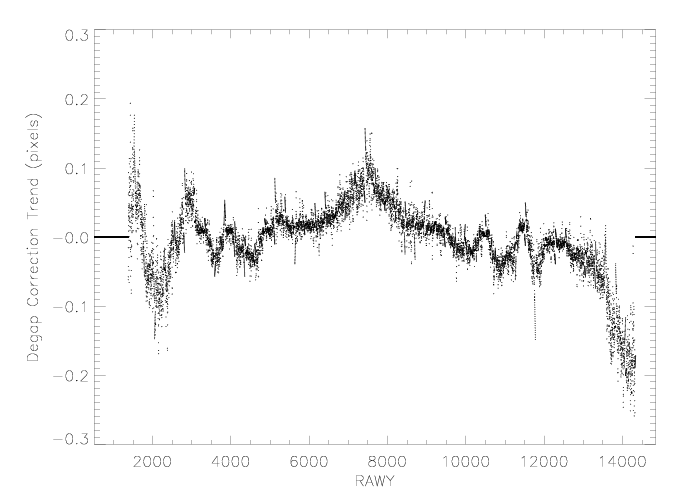# HRC-I Degap Update (2012)

The degap look-up calibration that was developed in 2007 suffers from a bias to larger than necessary correction near the gap boundaries. This is illustrated in the figure below which shows the data, information used to derive the degap values and degap values near one of the edges of CRSU = 30. The degap look-up values are determined by calculating the source position on the detector for each event and then determining the mean of the RAW position for a given modeled position with selected coarse position (e.g. CRSU) and AMP_SF value. As the modeled position nears the gap, the deviation is biased to a larger correction as event begin to appear on the adjacent tap.Figure 1:Example of the bias in the derived degap look-up values near a gap boundary (dashed vertical line). Dots are from the events from Capella that form the basis of the determination. The red error-bars show the mean deviations of events at a given modeled position re-cast into the RAW space. The solid black curve is the degap look-up derived from the red-points. The solid blue curve is the update described here.

In the development of the degap look-up values for near aimpoint region the HRC-S, I explored improvements to the derivation of the values in this near-gap region so as to remove or reduce the bias. First, the normal algorithm is used to determine the degap look-up values. Then the values within a pad-region relative to the gap are reset to values determined from a linear extrapolation from a region of the same width just inside the pad. The blue curve in figure 1 is the result of applying this modification.

Most of the degap look-up values are not affected by this change so the improvement to image quality is small. The most apparent way to see the improvement is to plot the source events in CHIP coordinates. Figure 2 shows such a comparison using data from ObsID 13182 (AR Lac). The bright straight horizontal and vertical streaks in the left pane are at the gap locations and are caused by the biased degap correction. The streaks are strongly suppressed in the right pane.Figure 21: CHIP coordinate comparison of AR Lac source events from ObsID 13182. Left pane uses degap corrections derived in 2007 and that are biased near the gap regions. Right pane uses a new degap that has the near-gap corrections modified.

I performed a few iterations of the degap derivation with this modification with the expectation that there would be minimal changes other than at the near-gap regions. However, In comparing the degap look-up values between iterations systematic trends are apparent. Figure3 shows these trends for the two axes. The magnitude of the trend is small but the trend is additive, each iteration grows the correction that will be applied.Figure 3: Trend in the derived degap correction between iterations.

This systematic trend is a symptom that will occur if the nominal HRC coordinates of the telescope aimpoint calculated in the algorithm as the SIM is translated among the observations is slightly off. The algorithm assumes perfect linear translation of the SIM and the deviations from this are reflected in the DY, DZ, and DTHETA columns of the aspect solution. The magnitude of the trends (≤ ~0.2 pixels or ~1.3 microns) are smaller than the uncertainties on the aspect solution DY and DZ, The trends may simply reflect this error in the actual location of the aimpoint on the HRC for each observation.

The updated degap look-up table is here: hrci_gaplookup_test_d.fits